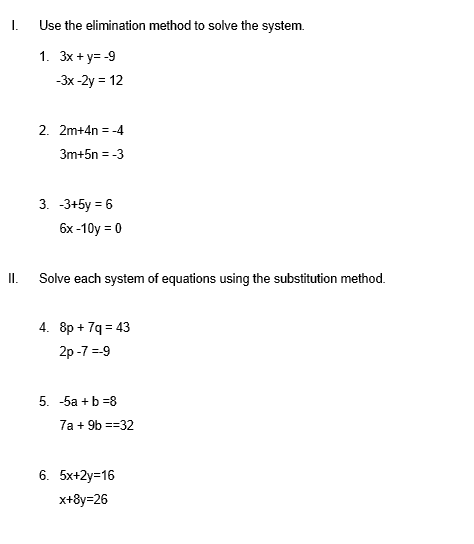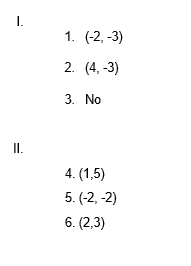# System of Equations Worksheet (with answer key + PDF)

A system of equations is a group or arrangement of equations that are handled jointly. Both algebraic and graphic methods can be used to solve these equations. The intersection of two lines is the location where the system of equations has a solution.

## What is the “System of Equations Worksheet”?

This worksheet will explore some of the systems of equations.

## What are the system equations?

The term “system of equations” or “equation system” in mathematics refers to a finite set of equations for which common solutions are sought. A set of simultaneous equations is also known as a system of equations or an equation system.

This worksheet will help the students to understand the system of equations. With the aid of the examples below, let’s learn how to recognize linear equations.

## Instructions on how to use the “System of Equations Worksheet .”

Study the concept and examples given and try to solve the given exercises below.

## Conclusion

When there are multiple unknowns and there is sufficient information to set up equations in those unknowns, systems of equations are used to solve applications. In general, if there are n unknowns, we need enough details to construct n equations in those unknowns.

If you have any inquiries or feedback, please let us know.

## System of Equations Worksheet (with answer key + PDF)

System of Linear Equations Solutions

A linear equation is one that has one or more variables and produces a straight line when plotted. The term “system of linear equations” refers to a set of two or more linear equations with at least two variables each, all of which are taken into account simultaneously.

Solution Type:

1. A unique solution exists if a numerical value for each variable is found that will satisfy the system of equations.
1.  Some linear equations may not have a solution or have infinitely many solutions.
1.  A consistent system of equations will have at least 1 solution whereas a system with no solution is an inconsistent system.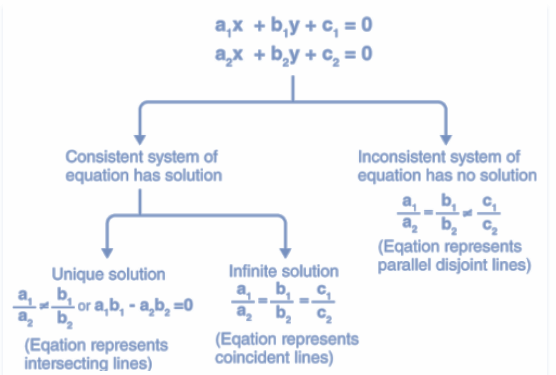Methods To Solve System of Equations

• Substitution Method: The method of substitution is more precise when locating the answer to a system of linear equations with decimal or fraction values. In order to find the value of the second variable and solve the given system, one variable must first be solved before its value is plugged into the second equation. Check the answers to both equations after that.
• Elimination Method: The addition method is another name for it. The sum of the two terms with the same variable is 0 when added to the opposite coefficients. Not all systems, though, will have two terms for a single variable with diametrically opposed coefficients. To remove one of the variables, one or both equations are then multiplied by the other.

Examples Of System of Equations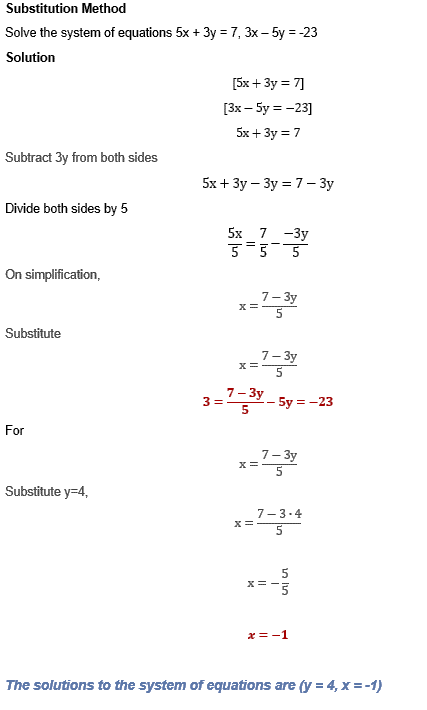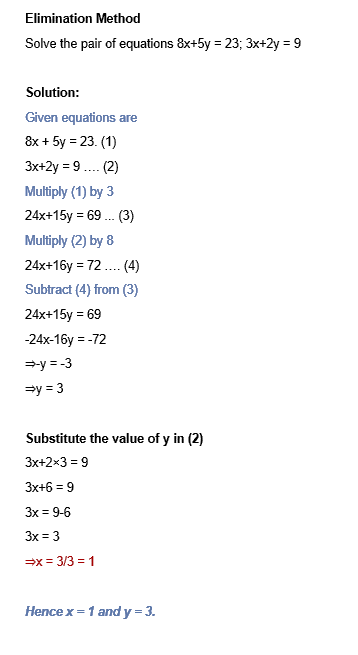## Worksheet